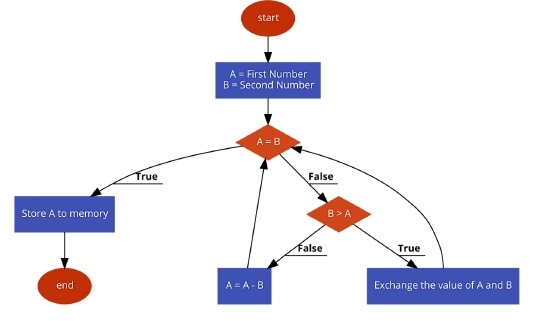# Program to find the HCF of two given bytes in 8085 Microprocessor

Here we will see how to find the HCF or GCD of two given bytes using 8085. The numbers are 8-bit numbers, nor larger than that.

Problem Statement

Write an 8085 Assembly language program to find the HCF or GCD of two numbers stored at memory location 8000H and 8001H.

Discussion

Here we will use the Euclidean algorithm to find HCF. This algorithm is very simple. We have to follow these steps−

• If first number and second number are same, then
a) go to step 3.

Else if first number < second number, the
b) exchange no1 and no2.

• first-number ← first-number – second-number; go to step 1
• Display result as first-number

The values are stored at location 8000H and 8001H, and the result will be stored at location 8050H.

First Input

Data

8000
2D
8001
69

8050
0F

Second Input

Data

8000
25
8001
35

8050
01

## Flow Diagram## Program

HEX Codes
Labels
Mnemonics
F000
21, 00, 80

LXI H,8000H
Point to the first number
F003
7E

MOV A,M
Load the first number into Acc
F004
23

INX H
Point to next location
F005
46

MOV B,M
F006
B8
LOOP
CMP B
Compare B with A
F007
CA, 17, F0

JZ STORE
When A and B are same, store the result
F00A
DA, 11, F0

JC EXG
If B > A, then exchange B and A
F00D
90

SUB B
if B < A, subtract B from A
F00E
C3, 06, F0

JMP LOOP
F011
48
EXG
MOV C,B
F012
47

MOV B,A
Move A to B
F013
79

MOV A,C
Move C to A
F014
C3, 06, F0

JMP LOOP
F017
32, 50, 80
STORE
STA 8050H
Store the value into memory
F01A
76

HLT
Terminate the program

## Output

First Output

Data

8050
0F

Second Output

Data

8050
01

Updated on: 06-Jul-2020

305 Views